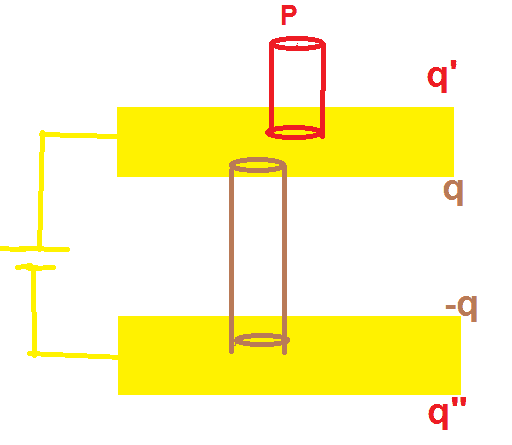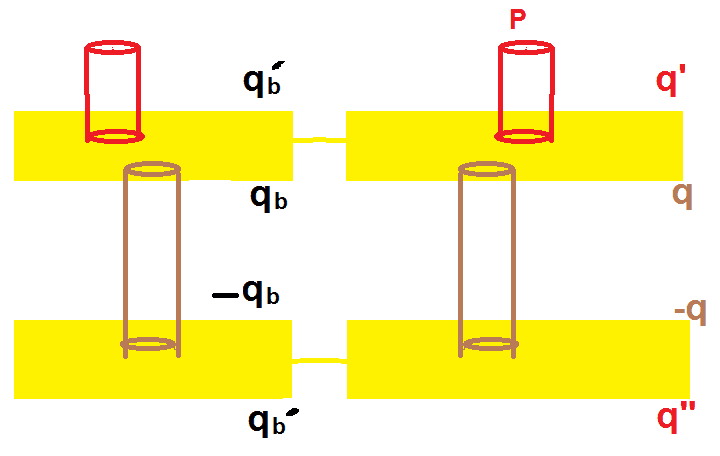# Charge upon a parallel plate capacitor

Pushoam
Homework Statement:
Choose the correct options.
Each plate of a parallel plate capacitor has a charge Q on it. The capacitor is now connected to a battery. Now,
a) the facing surfaces of the capacitor have equal and opposite charges.
b) the two plates of the capacitor have equal and opposite charges.
c)the battery supplies equal and opposite charges to the two plates.
d) the outer surface of the plates have equal charges.
Relevant Equations:
Gauss's law: $$\oint \vec E\cdot d\vec A= \frac{ q_{en}} {\epsilon_0}$$
a) if I take a Gaussian cylindrical surface whose circular area are present in the meat of the two plates of the capacitor, then the electric flux through this Gaussian surface is zero ( as the electric field inside the meatof the capacitor is zero and between the capacitors, electric field is perpendicular to the cylindrical surface).
So, charge enclosed by the Gaussian surface is zero.Hence the two facing surface of the capacitor have equal and opposite charges.

b) each plate of the conductor is equipotential. Hence the surface charge density on the inner surface is equal to that on the outer surface. Hence the two plates of the capacitor have equal and opposite charges.
c) to hold b) true the battery has to provide unqual and opposite charges to the two plates.
d) the outer surface charges of the plates have to be opposite.

Homework Helper
Gold Member
2022 Award
Each plate of a parallel plate capacitor has a charge Q on it.
I take it that is intentional, that they don't just have the same magnitude of charge but also the same sign of charge.
Is that what you assumed?

Pushoam
I take it that is intentional, that they don't just have the same magnitude of charge but also the same sign of charge.
Is that what you assumed?
Yes, initially both the plates have charge Q (same sign). Then, the capacitor is connected to the battery.

Homework Helper
Gold Member
2022 Award
Yes, initially both the plates have charge Q (same sign). Then, the capacitor is connected to the battery.
Why don't your arguments for a) and b) apply before the plates are connected to the battery?
It might help to consider what a battery is similar to. Is it similar to a simple conductor? To a charged capacitor?

Pushoam
Why don't your arguments for a) and b) apply before the plates are connected to the battery?
It might help to consider what a battery is similar to. Is it similar to a simple conductor? To a charged capacitor?
Before connecting to the battery, the plates are given charge Q as per question.
The battery is a device which maintains a given potential difference.

Is is true that the total charge supplied by a battery is always 0, i.e. if it gives Q to one plate, then it gives - Q to another plate?

Last edited:
Homework Helper
Gold Member
2022 Award
The battery is a device which maintains a given potential difference.
Yes.
the total charge supplied by a battery is always 0
That I'm less sure of. Supercapacitors are used as batteries. What would happen if, instead of a battery, a capacitor with charges +/-q were attached?

Pushoam
What would happen if, instead of a battery, a capacitor with charges +/-q were attached?
The total charge supplied by the capacitor (as battery) is 0 as it gives charge q from the positive plate and -q from the negative plate.

Homework Helper
Gold Member
2022 Award
The total charge supplied by the capacitor (as battery) is 0 as it gives charge q from the positive plate and -q from the negative plate.
So you'd have two capacitors in parallel, with one having a net zero charge but the other having a net charge of 2Q?

PushoamTaking the brown cylindrical surface as the Gaussian surface and applying the Gauss's law and considering the fact that electric field inside the material of conductor is 0 gives that surface charge densities on the facing surfaces are equal and opposite. Hence, the charges on the facing surfaces are q and -q.

Now, for outer surface, Electric field at P is calculated first using the Gaussian surface and applying Gauss's law and then, due to all the charged surfaces individually and then the two expressions are equated. $$\frac {q'}{A\epsilon_0} = \frac {q'}{2A\epsilon_0} +\frac {q}{2A\epsilon_0} +\frac {-q}{2A\epsilon_0} +\frac {q"}{2A\epsilon_0}$$ $$q' =q"$$

Hence, the inner surface charges of both plates are equal and opposite and the outer surface charges of both plates are equal and same.

The battery doesn't supply the charges. It creates the potential difference across the plates. As a result, electrons of plate at higher potential move towards plate at lower potential creating inner surface charge q and -q.

Now, the conservation of charge gives, $$q' +q -q+q' = 2Q \Rightarrow q' =Q$$

Hence, the correct options are a, c and d.

Last edited:
Homework Helper
Gold Member
2022 Award
View attachment 321947
Taking the brown cylindrical surface as the Gaussian surface and applying the Gauss's law and considering the fact that electric field inside the material of conductor is 0 gives that surface charge densities on the facing surfaces are equal and opposite. Hence, the charges on the facing surfaces are q and -q.

Now, for outer surface, Electric field at P is calculated first using the Gaussian surface and applying Gauss's law and then, due to all the charged surfaces individually and then the two expressions are equated. $$\frac {q'}{A\epsilon_0} = \frac {q'}{2A\epsilon_0} +\frac {q}{2A\epsilon_0} +\frac {-q}{2A\epsilon_0} +\frac {q"}{2A\epsilon_0}$$ $$q' =q"$$

Hence, the inner surface charges of both plates are equal and opposite and the outer surface charges of both plates are equal and same.

The battery doesn't supply the charges. It creates the potential difference across the plates. As a result, electrons of plate at higher potential move towards plate at lower potential creating inner surface charge q and -q.

Now, the conservation of charge gives, $$q' +q -q+q' = 2Q \Rightarrow q' =Q$$

Hence, the correct options are a, c and d.
I agree with your answers, but it is not clear that ##q'=Q##.
If we think of the battery as a charged capacitor, it would acquire some of the Q on each side, and now be carrying a net charge.

•Pushoam
Pushoam
I agree with your answers, but it is not clear that ##q'=Q##.
If we think of the battery as a charged capacitor, it would acquire some of the Q on each side, and now be carrying a net charge.
Let's consider the battery as a charged parallel plate capacitor. Then, the opposite charges are on the facing surfaces.
Now, if I draw a cylindrical Gaussian surface inside the capacitor such that its circular surfaces are in the material of the plates and cylindrical surface in the middle. Then, applying Gauss's law, one gets that the charges on the facing surfaces are opposite and equal, proving that the net charge is 0.

Homework Helper
Gold Member
2022 Award
Let's consider the battery as a charged parallel plate capacitor. Then, the opposite charges are on the facing surfaces.
Now, if I draw a cylindrical Gaussian surface inside the capacitor such that its circular surfaces are in the material of the plates and cylindrical surface in the middle. Then, applying Gauss's law, one gets that the charges on the facing surfaces are opposite and equal, proving that the net charge is 0.
If I connect a capacitor C1 with Q on each plate in parallel with uncharged capacitor C2, how will the charge redistribute? You seem to be claiming it won’t.

Last edited:
Homework Helper
View attachment 321947

Now, the conservation of charge gives, $$q' +q -q+q' = 2Q \Rightarrow q' =Q$$

Hence, the correct options are a, c and d.
For a capacitor connected to a battery the conservation of charge on the capacitor is not granted and not required.
You showed that the charges on the inner faces are opposite and equal. Also that q'=q''.
But the fact that these last two are equal to Q does not follow. I am not saying it cannot be true, but you need something else to prove it, if true.

•Pushoam
Pushoam
If I connect a capacitor C1 with Q on each plate in parallel with uncharged capacitor C2, how will the charge redistribute? You seem to be claiming it won’t.
If we think of the battery as a charged capacitor, it would acquire some of the Q on each side, and now be carrying a net charge.Let's say that the battery capacitor has capacitance Cb, initial charge Qb, and potential difference Vb and the capacitor has charge Q on each plate.
After the connection and coming to equilibrium, the system has the charge configuration given in the figure and potential difference V'b.
Calculations similar to those in post #9 show that outer surface charges on the plates of battery are same.

Now, $$q_b = C_b V'_b ~~\text{and}~~q = CV'_b$$ $$\Rightarrow q = \frac {q_b C}{C_b} ~~\text{and} ~~q_b = \frac{Q_B}{1+\frac C {C_B}}$$

So, after the connection, potential difference and facing surface charges of each plate changes.

The charge Q, too, redistributes on the outer surface of all plates as the connecting plates of the battery and capacitor are equipotential. Could you help me in finding the distribution?

On the other hand, an ideal battery is supposed to maintain the potential difference. Here, the potential difference changes. So, the capacitor battery is not an ideal battery.

Homework Helper
Gold Member
2022 Award
On the other hand, an ideal battery is supposed to maintain the potential difference
If Q' out of the initial Q on each plate moves into the battery, the potential difference between the plates is unaffected by that.

•nasu and Pushoam
Pushoam
If Q' out of the initial Q on each plate moves into the battery, the potential difference between the plates is unaffected by that.
This I have understood.
The thing is I am not able to prove that this Q' is nonzero. I just know that the charge will distribute bcause these plates are conducting.

Homework Helper
Gold Member
2022 Award
This I have understood.
The thing is I am not able to prove that this Q' is nonzero. I just know that the charge will distribute bcause these plates are conducting.
It will be nonzero if the battery acts at least a tiny bit like a capacitor.

Pushoam
It will be nonzero if the battery acts at least a tiny bit like a capacitor.
How can this be shown mathematically?

•